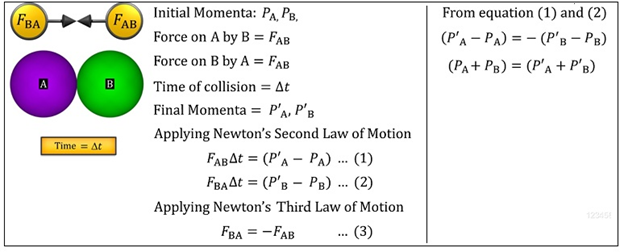Notes On Conservation of Momentum and Equilibrium of a Particle - CBSE Class 11 Physics
The Law of Conservation of Momentum states that the total momentum of an isolated system of interacting particles is conserved.  For an isolated system, there are no external forces acting on it.  Let us apply this law to the direct collision between two bodies A and B.This shows that the total momentum before the collision is equal to the total momentum after the collision.   Equilibrium of a Particle:  When the net external force acting on a particle is zero, the particle is said to be in equilibrium.  Applying the Newton’s First Law of Motion to this situation, we can say that the particle is either at rest or in uniform motion. When the particle is at rest, we say that the particle is in static equilibrium and when the body has uniform motion, we say that the particle is in dynamic equilibrium.   When two forces act on a particle and keep it in equilibrium, then both forces must be equal in magnitude but opposite in direction and the two forces must be collinear.  When three forces act on a particle and keep it in equilibrium, the three forces must be coplanar.  The resultant of any two forces is equal and opposite to the third force. This third force is called the equilibrant force.  The vector sum of the three forces must be equal to zero. When n number of forces act on a particle and keep it in equilibrium, the vector sum of the n forces must be equal to zero.

#### Summary

The Law of Conservation of Momentum states that the total momentum of an isolated system of interacting particles is conserved.  For an isolated system, there are no external forces acting on it.  Let us apply this law to the direct collision between two bodies A and B.This shows that the total momentum before the collision is equal to the total momentum after the collision.   Equilibrium of a Particle:  When the net external force acting on a particle is zero, the particle is said to be in equilibrium.  Applying the Newton’s First Law of Motion to this situation, we can say that the particle is either at rest or in uniform motion. When the particle is at rest, we say that the particle is in static equilibrium and when the body has uniform motion, we say that the particle is in dynamic equilibrium.   When two forces act on a particle and keep it in equilibrium, then both forces must be equal in magnitude but opposite in direction and the two forces must be collinear.  When three forces act on a particle and keep it in equilibrium, the three forces must be coplanar.  The resultant of any two forces is equal and opposite to the third force. This third force is called the equilibrant force.  The vector sum of the three forces must be equal to zero. When n number of forces act on a particle and keep it in equilibrium, the vector sum of the n forces must be equal to zero.

Previous